# One TTS Alignment to Rule Them All

Published:## Overview

In our recent paper, we present an unsupervised alignment learning framework that can learn speech-text alignments online in TTS models. It combines the forward-sum algorithm, the Viterbi algorithm, and a simple and efficient static prior. We demonstrate that TTS alignments can be learnt entirely online and following are the key highlights of our work:

• The alignment learning framework eliminates the need for forced aligners which are expensive to use and often not readily available for certain languages
• Human evaluation using pairwise test indicates improvements in overall speech quality
• Alignment framework results in much less alignment errors on long utterances and faster convergence
• The alignment framework is readily applicable to various TTS architectures - both autoregressive as well as non autoregressive TTS models

## Method

Below diagram illustrates the Alignment Learning Framework architecture: autoregressive models use a sequential attention mechanism to generate alignments between text and speech (mels). Non-autoregressive models encode text and speech (mels) using simple 1D convolutions and use pairwise L2 distance to compute the alignments.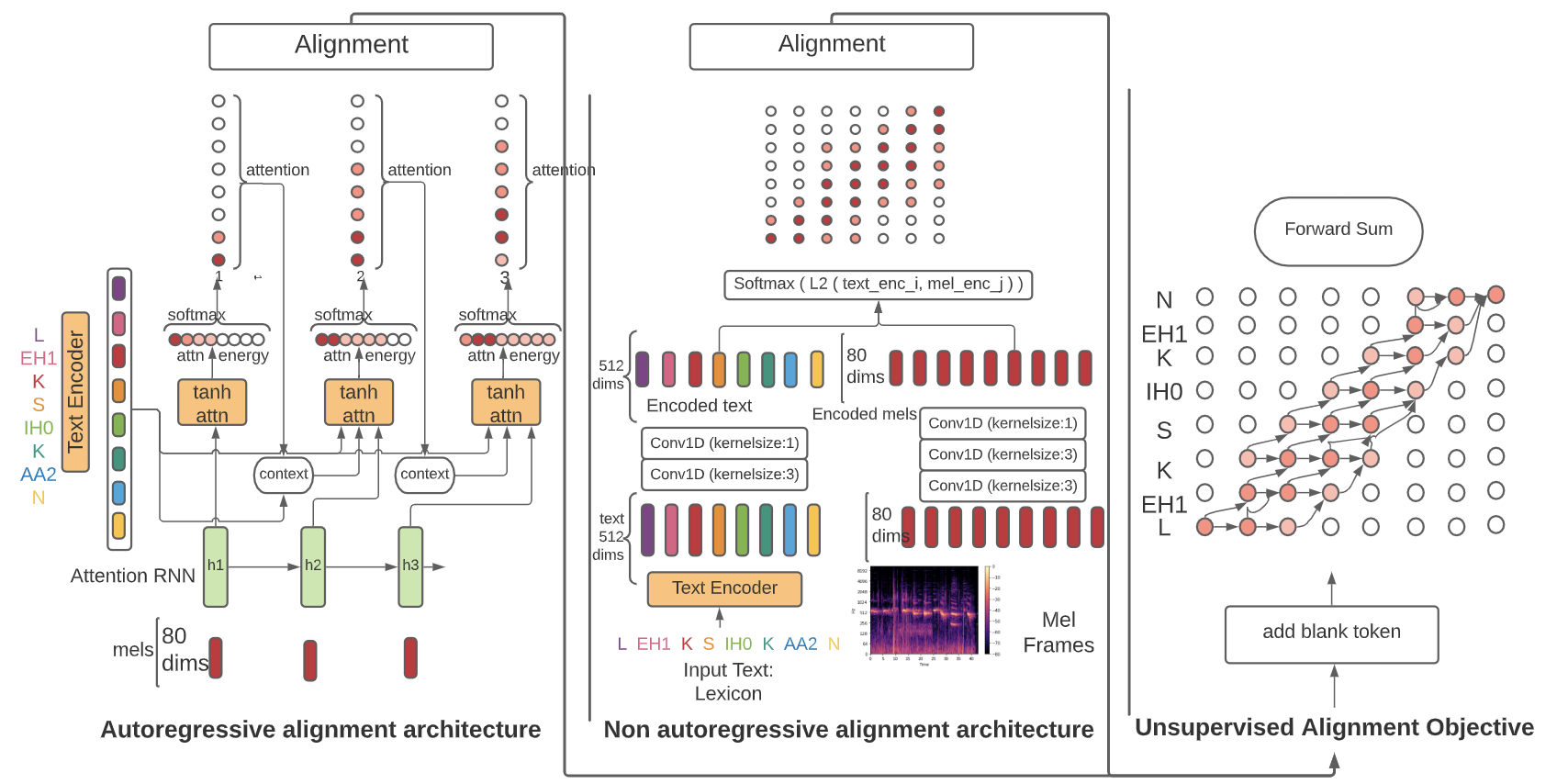To learn these alignments, we optimize the following objective that maximizes the probability of text given mel-spectrograms using the forward-sum algorithm used in Hidden Markov Models (HMMs).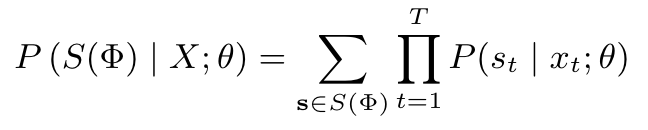where:

's' a specific alignment between mel-spectrograms and text (eg:s1=φ1,s2 =φ1,s3 =φ2,...,sT=φN),

S(Φ) is the set of all possible valid monotonic alignments,

P(s_t|x_t) is the likelihood of a specific text tokenst=φ_i aligned for melframe x_t at timestep t.


As it can be observed, this objective sums over all possible alignments. In the RAD-TTS appendix, we demonstrate this objective is equivalent to minimizing the CTC loss when we constrain it to monotonic alignments. CTC loss is widely used in the Automatic Speech Recognition, and we use an efficient implementation of CTC available in Pytorch.

Following is an example of a learnt soft speech text alignment using our alignment learning framework.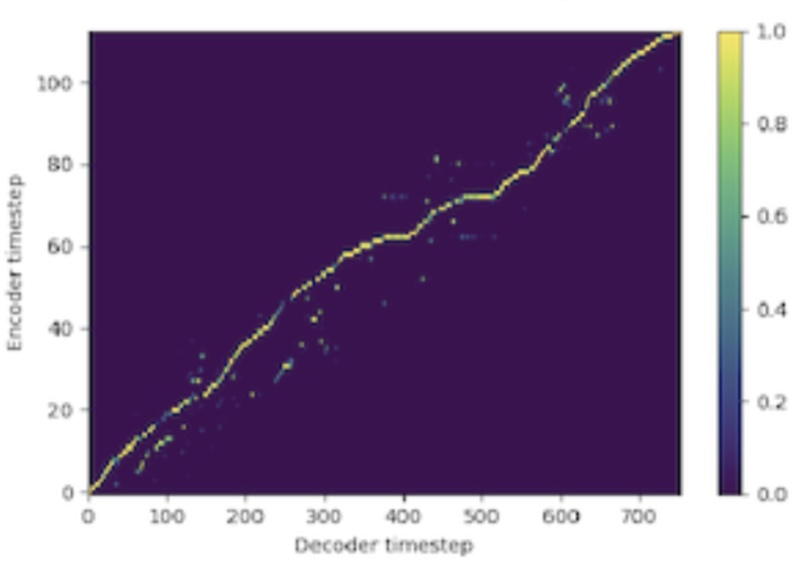For autoregressive models, we use the above objective alongside the standard decoder objective to learn alignments.

Non-autoregressive models have durations factored out and hence require number of output samples/phoneme. We use the Viterbi Algorithm to convert soft alignments to binarized alignments representing the most likely monotonic path. The following figure shows the most likely path extracted using Viterbi algorithm over the soft alignment shown above.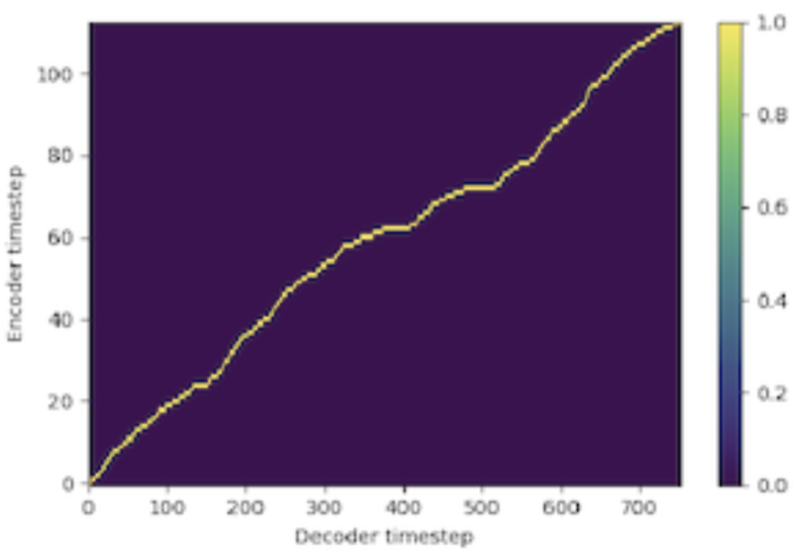## Results and Samples

We evaluate the proposed alignment framework over both autoregressive (Flowtron, Tacotron2) and non-autoregressive models (FastSpeech2, RAD-TTS, FastPitch). We gave the human evaluators an anonymous preference test to choose their preferred sample. The listeners were shown the text and asked to select samples with the best overall quality, defined by accuracy of text, its pleasantness and naturalness. Following table summarizes the results.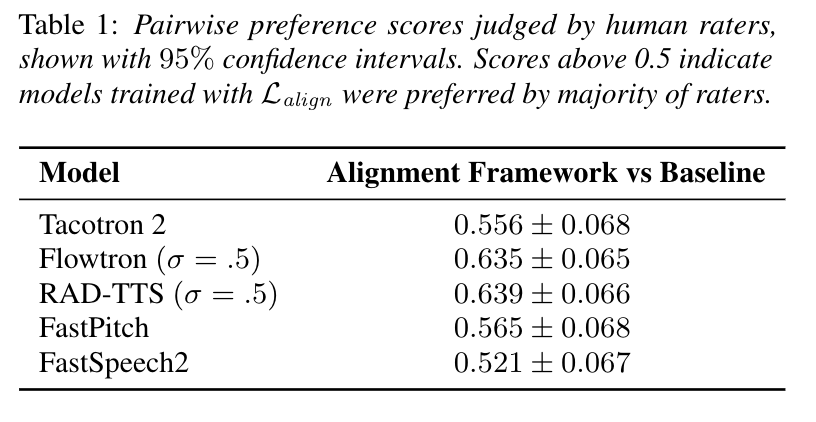### Samples comparing baseline models vs Alignment Framework Samples

The following table provides the samples comparing with baselines:

Flowtron baselineFlowtron with alignment framework
Tacotron2 baselineTacotron2 with alignment framework
FastPitch baseline with external Tacotron2 durationsFastPitch with alignment framework
FastSpeech2 baselineFastSpeech2 with alignment framework

Full list of samples can be found here.

### Evaluation over long input prompts

We measure character error rate (CER) between synthesized and input texts using an external speech recognition model to evaluate the robustness of the alignments on long utterances. Figure below shows that autoregressive models withLalignhave a lower CER, providing evidence thatthe alignment objective results in more robust speech for long utterances.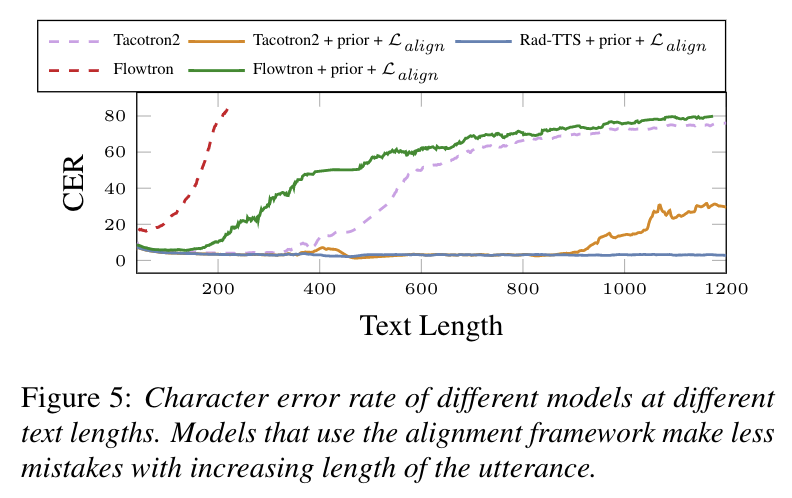The following table demonstrates synthesized samples for long prompts from a Flowtron baseline model compared to one that uses alignment framework:

Flowtron baselineFlowtron with alignment framework

Baseline Flowtron Alignment: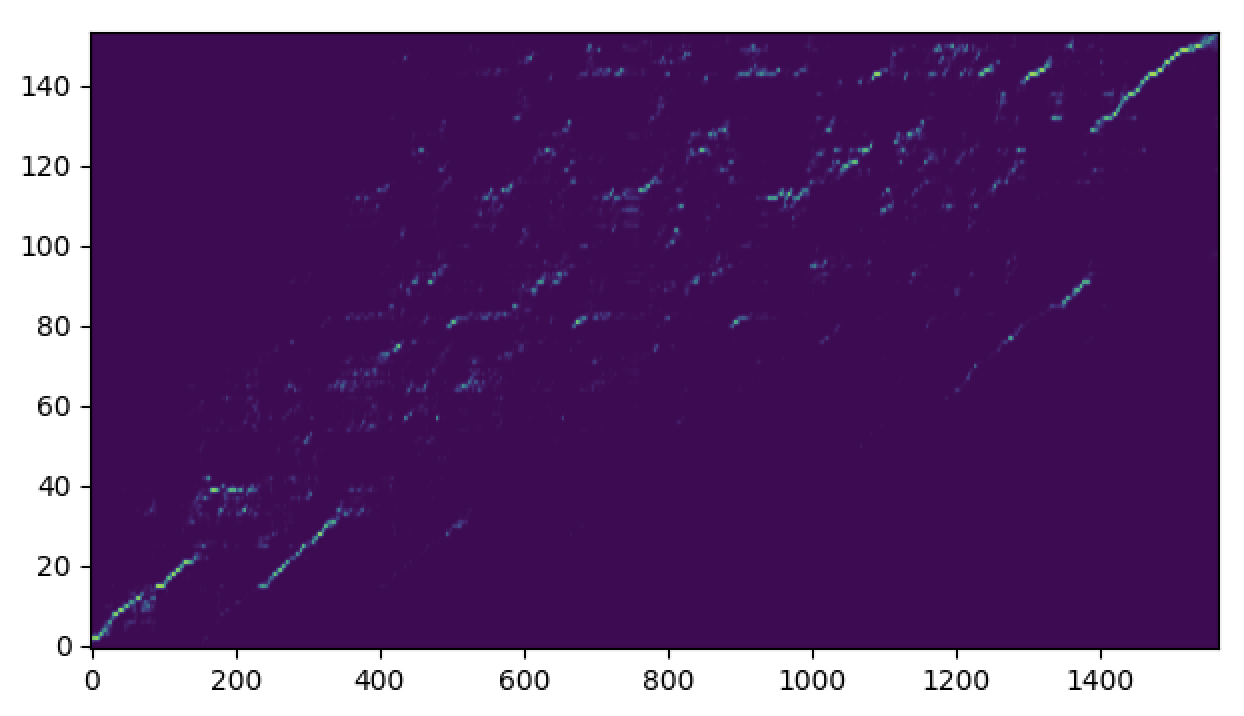Alignment Framework Flowtron Alignment: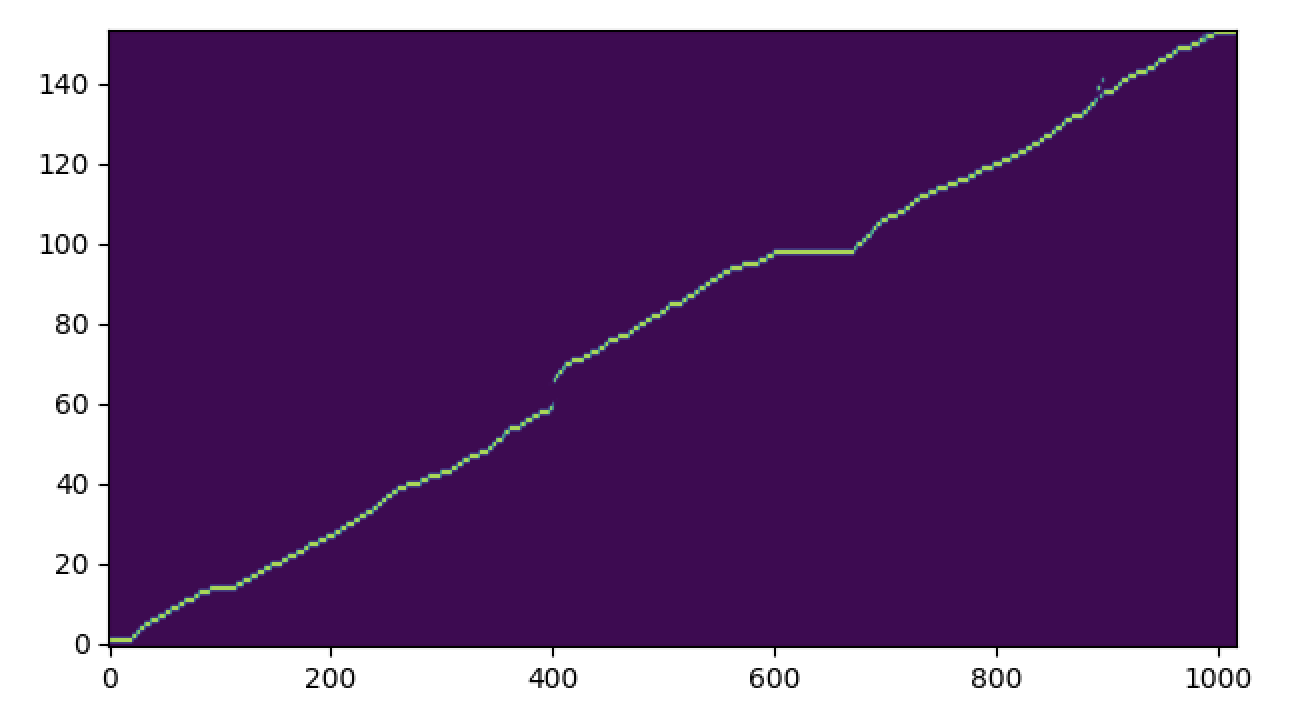As we reduce the character length, baseline flowtron sounds closer to the alignment framework version. This is because for shorter prompts, its easier to get the alignments. However, we do observe that even on shorter sentences, the alignment framework version is much more clearer than baseline:

Flowtron baselineFlowtron with alignment framework

### Scaling of alignment framework to new languages

In this section, we demonstrate that the alignment framework that we propose can be applied directly to a new language like Spanish without any changes. Generally, most parallel TTS systems until now depend on external aligners to extract token/phoneme durations. This requires the external aligner to be available in the new language. However, our alignment framework is language agnostic and can be applied to any new language without any dependency or extra effort.

We train our RAD-TTS model over Spanish data using the IPA (international phonetic alphabet). Due to the alignment framework, we’re able to train the model without any changes. Following is a couple of samples from our RAD-TTS model in Spanish:

## Implementation details

Implementation of the alignment framework for autoregressive models such as Flowtron can be found at Flowtron Implementation. Reference implementation for parallel models can be found at Talknet implementation. More implementations coming soon.

The following pseudo code further elaborates the implementation of the alignment loss:

class ForwardSumLoss(torch.nn.Module):
def __init__(self, blank_logprob=-1):
super(ForwardSumLoss, self).__init__()
self.log_softmax = torch.nn.LogSoftmax(dim=3)
self.blank_logprob = blank_logprob
self.CTCLoss = nn.CTCLoss(zero_infinity=True)

def forward(self, attn_logprob, text_lens, mel_lens):
"""
Args:
attn_logprob: batch x 1 x max(mel_lens) x max(text_lens)
batched tensor of attention log
of longest sequence in each dimension
text_lens: batch-D vector of length of
each text sequence
mel_lens: batch-D vector of length of
each mel sequence
"""
# The CTC loss module assumes the existence of a blank token
# that can be optionally inserted anywhere in the sequence for
# a fixed probability.
# A row must be added to the attention matrix to account for this
pad=(1, 0, 0, 0, 0, 0, 0, 0),
value=self.blank_logprob)
cost_total = 0.0
# for-loop over batch because of variable-length
# sequences
for bid in range(attn_logprob.shape):
# construct the target sequence. Every
# text token is mapped to a unique sequence number,
# thereby ensuring the monotonicity constraint
target_seq = torch.arange(1, text_lens[bid]+1)
target_seq=target_seq.unsqueeze(0)
curr_logprob = attn_logprob_pd[bid].permute(1, 0, 2)
curr_log_prob = curr_logprob[:mel_lens[bid],:,:text_lens[bid]+1]
curr_logprob = self.log_softmax(curr_logprob[None])
cost = self.CTCLoss(curr_logprob,
target_seq,
input_lengths=mel_lens[bid:bid+1],
target_lengths=text_lens[bid:bid+1])
cost_total += cost
# average cost over batch
cost_total = cost_total/attn_logprob.shape
return cost_total


## Citation

@misc{badlani2021tts,
title={One TTS Alignment To Rule Them All},
author={Rohan Badlani and Adrian Łancucki and Kevin J. Shih and Rafael Valle and Wei Ping and Bryan Catanzaro},
year={2021},
eprint={2108.10447},
archivePrefix={arXiv},
primaryClass={cs.SD}
}